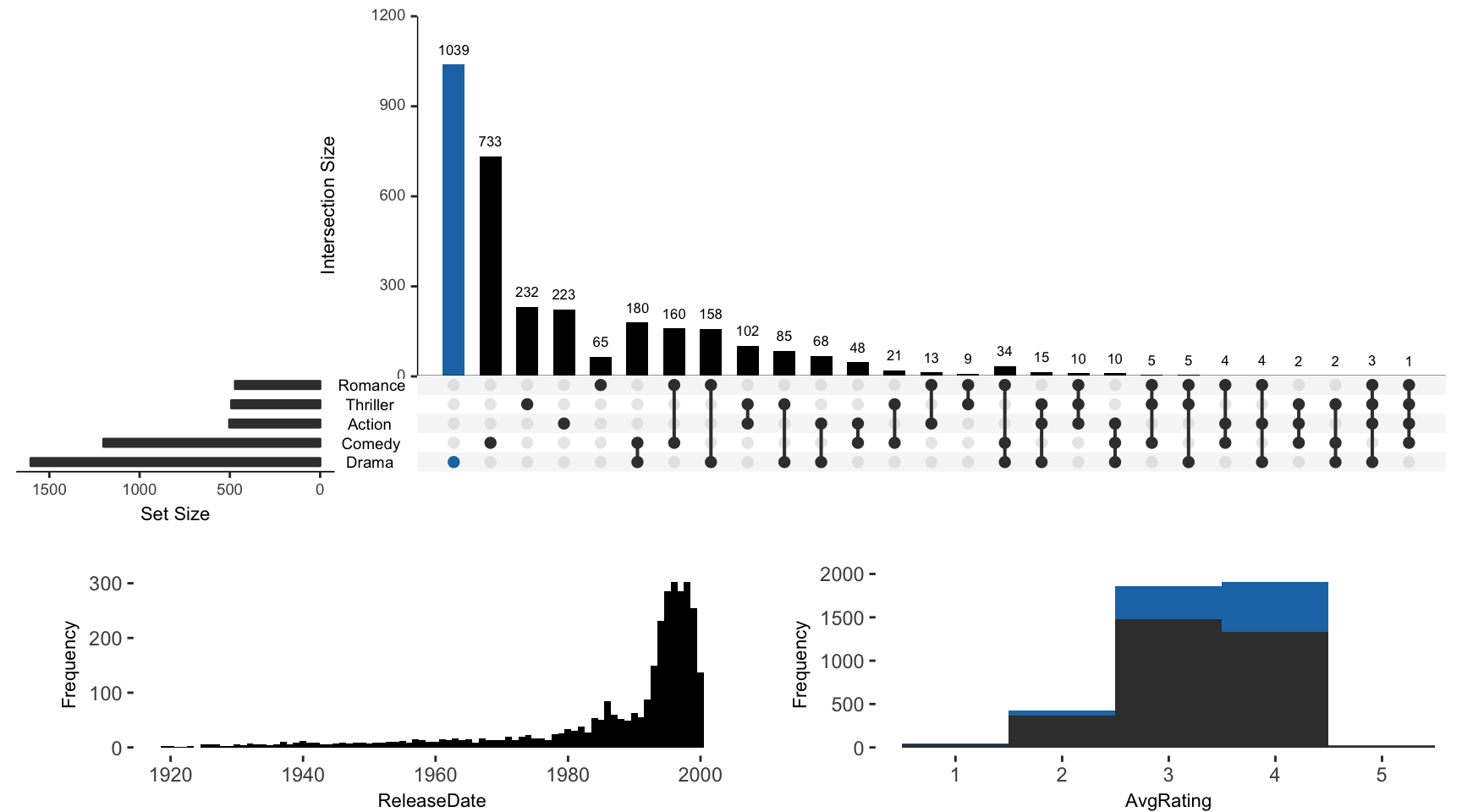For all examples the movies data set contained in the package will be used.

library(UpSetR)
library(ggplot2)
## Warning: package 'ggplot2' was built under R version 3.4.4
library(grid)
library(plyr)
movies <- read.csv(system.file("extdata", "movies.csv", package = "UpSetR"),
header = T, sep = ";")

attribute.plots Parameter Breakdown

The attribute.plots parameter is broken down into 3 fields: gridrows, plots, and ncols

• gridrows: specifies how much to expand the plot window to add room for attribute plots. The UpSetR plot is plotted on a 100 by 100 grid. So for example, if we set gridrows to 50, the new grid layout would be 150 by 100, setting aside 1/3 of the plot for the attribute plots.

• plots: takes a list of paramters. These paramters include plot, x, y (if applicable), and queries.

• plot: is a function that returns a ggplot

• x: is the x aesthetic to be used in the ggplot (entered as string)

• y: is the y aesthetic to be used in the ggplot (entered as string)

• queries: indicates whether or not to overlay the plot with the queries present. If queries is TRUE, the attribute plot will be overlayed with data from the queries. If queries is FALSE, no query results will be plotted on the attribute plot.

• ncols: specifies how the plots should be arranged in the gridrows space. If two attribute plots are entered and ncols is 1,then the plots will display one above the other. Alternatively, if two attribute plots are entered and ncols is 2, the attribute plots will be displayed side by side.

Additional: to add a legend of the queries, use query.legend = "bottom" (see Example 2).

Example 1: Built-In Attribute Histogram

Example of how to add built-in histogram attribute plot. If main.bar.color is not specified as black, elements contained in black intersection size bars will be represented as gray in attribute plots.

upset(movies, main.bar.color = "black", queries = list(list(query = intersects,
params = list("Drama"), active = T)), attribute.plots = list(gridrows = 50,
plots = list(list(plot = histogram, x = "ReleaseDate", queries = F), list(plot = histogram,
x = "AvgRating", queries = T)), ncols = 2))Example 2: Built-In Attribute Scatter Plot

Example of how to add built-in attribute scatter plot. If main.bar.color not specified as black, elements contained in black intersection size bars will be represented as gray in attribute plots.

notice the use of query.legend

upset(movies, main.bar.color = "black", queries = list(list(query = intersects,
params = list("Drama"), color = "red", active = F), list(query = intersects,
params = list("Action", "Drama"), active = T), list(query = intersects,
params = list("Drama", "Comedy", "Action"), color = "orange", active = T)),
attribute.plots = list(gridrows = 45, plots = list(list(plot = scatter_plot,
x = "ReleaseDate", y = "AvgRating", queries = T), list(plot = scatter_plot,
x = "AvgRating", y = "Watches", queries = F)), ncols = 2), query.legend = "bottom")